# Coin Change 2 (DP – 22)

Problem Link: Ways to Make a Coin Change

We are given an array Arr with N distinct coins and a target. We have an infinite supply of each coin denomination. We need to find the number of ways we sum up the coin values to give us the target.

Each coin can be used any number of times.

```Example: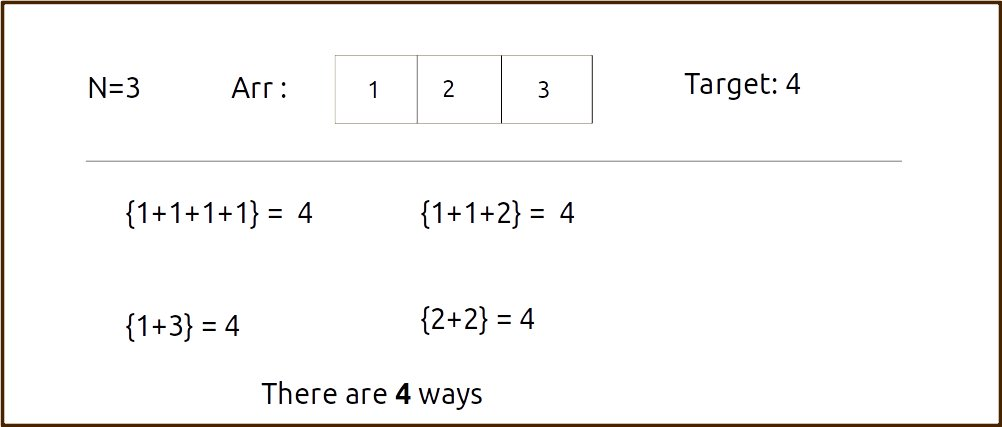```

Disclaimer: Don’t jump directly to the solution, try it out yourself first.

Pre-req: 0/1 Knapsack

### Solution :

Steps to form the recursive solution:

We will first form the recursive solution by the three points mentioned in Dynamic Programming Introduction

Step 1: Express the problem in terms of indexes.

We are given ‘n’ coins. Their denomination value is given by the array ‘arr’.So clearly one parameter will be ‘ind’, i.e index up to which the array items are being considered.

There is one more parameter, the given target value “T” which we want to achieve so that while generating subsequences, we can decide whether we want to include a particular coin or not.

So, we can say that initially, we need to find f(n-1, T) where T is the initial target given to us in the question. f(n-1, T) means we are finding the total number of ways to form the target T by considering coins from index 0 to index n-1 of the arr array.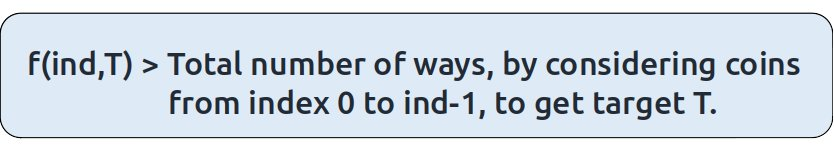Base Cases:

If ind==0, it means we are at the first item so we have only one coin denomination, therefore the following two cases can arise:

• T is divisible by arr  (eg: arr = 4 and T = 12)

In such a case where the target is divisible by the coin element value, we will return 1 as we will be able to form the target.

• T is not divisible by arr (eg: arr = 4 and T = 7)

In all other cases, we will not be able to form the target, so we will return 0.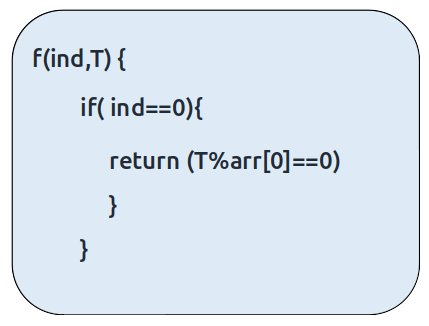Step 2: Try out all possible choices at a given index.

We need to generate all the subsequences. We will use the pick/non-pick technique as discussed in this video “Recursion on Subsequences”.

We have two choices:

• Exclude the current element in the subsequence: We first try to find a subsequence without considering the current index coin. If we exclude the current coin, the target sum will not be affected. So we will call the recursive function f(ind-1,T) to find the remaining answer.
• Include the current element in the subsequence: We will try to find a subsequence by considering the current icoin. As we have included the coin, the target sum will be updated to T-arr[ind].

Now here is the catch, as there is an unlimited supply of coins, we want to again form a solution with the same coin value. So we will not recursively call for f(ind-1, T-arr[ind]) rather we will stay at that index only and call for f(find, T-arr[ind]) to find the answer.
Note: We will consider the current coin only when its denomination value (arr[ind]) is less than or equal to the target T.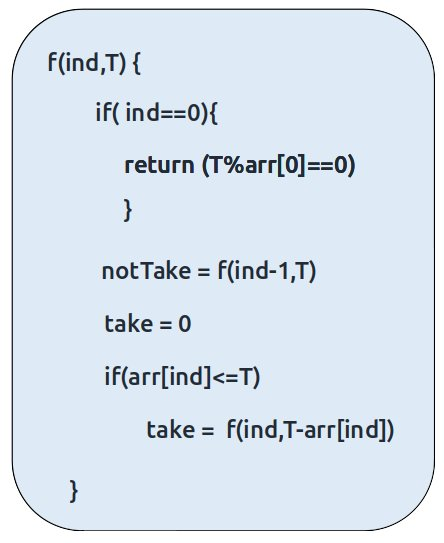Step 3:  Return the sum of take and notTake

As we have to return the total number of ways we can form the target, we will return the sum of notTake and take as our answer.

The final pseudocode after steps 1, 2, and 3: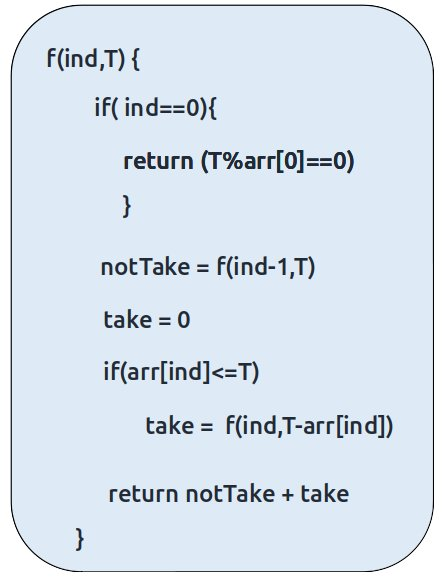Steps to memoize a recursive solution:

If we draw the recursion tree, we will see that there are overlapping subproblems. In order to convert a recursive solution to the following steps will be taken:

1. Create a dp array of size [n][T+1]. The size of the input array is ‘N’, so the index will always lie between ‘0’ and ‘n-1’. The target can take any value between ‘0’ and ‘T’. Therefore we take the dp array as dp[n][T+1]
2. We initialize the dp array to -1.
3. Whenever we want to find the answer of particular parameters (say f(ind,target)), we first check whether the answer is already calculated using the dp array(i.e dp[ind][target]!= -1 ). If yes, simply return the value from the dp array.
4. If not, then we are finding the answer for the given value for the first time, we will use the recursive relation as usual but before returning from the function, we will set dp[ind][target] to the solution we get.

Code:

## C++ Code

``````#include <bits/stdc++.h>

using namespace std;

long countWaysToMakeChangeUtil(vector<int>& arr,int ind, int T, vector<vector<long
>>& dp){

if(ind == 0){
return (T%arr==0);
}

if(dp[ind][T]!=-1)
return dp[ind][T];

long notTaken = countWaysToMakeChangeUtil(arr,ind-1,T,dp);

long taken = 0;
if(arr[ind] <= T)
taken = countWaysToMakeChangeUtil(arr,ind,T-arr[ind],dp);

return dp[ind][T] = notTaken + taken;
}

long countWaysToMakeChange(vector<int>& arr, int n, int T){

vector<vector<long>> dp(n,vector<long>(T+1,-1));
return countWaysToMakeChangeUtil(arr,n-1, T, dp);
}

int main() {

vector<int> arr ={1,2,3};
int target=4;

int n =arr.size();

cout<<"The total number of ways is " <<countWaysToMakeChange(arr,n,target);
}
``````

Output:

The total number of ways is 4

Time Complexity: O(N*T)

Reason: There are N*W states therefore at max ‘N*T’ new problems will be solved.

Space Complexity: O(N*T) + O(N)

Reason: We are using a recursion stack space(O(N)) and a 2D array ( O(N*T)).

## Java Code

``````import java.util.*;

class TUF{
static long countWaysToMakeChangeUtil(int[] arr,int ind, int T, long[][] dp){

if(ind == 0){
if(T%arr==0)
return 1;
else
return 0;
}

if(dp[ind][T]!=-1)
return dp[ind][T];

long notTaken = countWaysToMakeChangeUtil(arr,ind-1,T,dp);

long taken = 0;
if(arr[ind] <= T)
taken = countWaysToMakeChangeUtil(arr,ind,T-arr[ind],dp);

return dp[ind][T] = notTaken + taken;
}

static long countWaysToMakeChange(int[] arr, int n, int T){

long dp[][]=new long[n][T+1];
for(long row[]: dp)
Arrays.fill(row,-1);
return countWaysToMakeChangeUtil(arr,n-1, T, dp);
}

public static void main(String args[]) {

int arr[] ={1,2,3};
int target=4;

int n =arr.length;

System.out.println("The total number of ways is "+countWaysToMakeChange(arr,n,
target));
}
}``````

Output:

The total number of ways is 4

Time Complexity: O(N*T)

Reason: There are N*W states therefore at max ‘N*T’ new problems will be solved.

Space Complexity: O(N*T) + O(N)

Reason: We are using a recursion stack space(O(N)) and a 2D array ( O(N*T)).

Steps to convert Recursive Solution to Tabulation one.

To convert the memoization approach to a tabulation one, create a dp array with the same size as done in memoization. We can initialize it as 0.

First, we need to initialize the base conditions of the recursive solution.

• At ind==0, we are considering the first element, if the target value is divisible by the first coin’s value, we set the cell’s value as 1 else 0.
• Next, we are done for the first row, so our ‘ind’ variable will move from 1 to n-1, whereas our ‘target’ variable will move from 0 to ‘T’. We will set the nested loops to traverse the dp array.
• Inside the nested loops we will apply the recursive logic to find the answer of the cell.
• When the nested loop execution has ended, we will return dp[n-1][T] as our answer.

Code:

## C++ Code

``````#include <bits/stdc++.h>

using namespace std;

long countWaysToMakeChange(vector<int>& arr, int n, int T){

vector<vector<long>> dp(n,vector<long>(T+1,0));

//Initializing base condition
for(int i=0;i<=T;i++){
if(i%arr==0)
dp[i]=1;
// Else condition is automatically fulfilled,
// as dp array is initialized to zero
}

for(int ind=1; ind<n;ind++){
for(int target=0;target<=T;target++){
long notTaken = dp[ind-1][target];

long taken = 0;
if(arr[ind]<=target)
taken = dp[ind][target-arr[ind]];

dp[ind][target] = notTaken + taken;
}
}

return dp[n-1][T];
}

int main() {

vector<int> arr ={1,2,3};
int target=4;

int n =arr.size();

cout<<"The total number of ways is " <<countWaysToMakeChange(arr,n,target);
}
``````

Output:

The total number of ways is 4

Time Complexity: O(N*T)

Reason: There are two nested loops

Space Complexity: O(N*T)

Reason: We are using an external array of size ‘N*T’. Stack Space is eliminated.

## Java Code

``````import java.util.*;

class TUF{
static long countWaysToMakeChange(int[] arr, int n, int T){

long dp[][]=new long[n][T+1];

//Initializing base condition
for(int i=0;i<=T;i++){
if(i%arr==0)
dp[i]=1;
// Else condition is automatically fulfilled,
// as dp array is initialized to zero
}

for(int ind=1; ind<n;ind++){
for(int target=0;target<=T;target++){
long notTaken = dp[ind-1][target];

long taken = 0;
if(arr[ind]<=target)
taken = dp[ind][target-arr[ind]];

dp[ind][target] = notTaken + taken;
}
}

return dp[n-1][T];
}

public static void main(String args[]) {

int arr[] ={1,2,3};
int target=4;

int n =arr.length;

System.out.println("The total number of ways is "+countWaysToMakeChange(arr,n,
target));
}
}``````

Output:

The total number of ways is 4

Time Complexity: O(N*T)

Reason: There are two nested loops

Space Complexity: O(N*T)

Reason: We are using an external array of size ‘N*T’. Stack Space is eliminated.

Part 3: Space Optimization

If we closely look the relation,

dp[ind][target] =  dp[ind-1][target] ,dp[ind-1][target-arr[ind]]

We see that to calculate a value of a cell of the dp array, we need only the previous row values (say prev). So, we don’t need to store an entire array. Hence we can space optimize it.

Note: We first need to initialize the first row as we had done in the tabulation approach.

Code:

## C++ Code

``````#include <bits/stdc++.h>

using namespace std;

long countWaysToMakeChange(vector<int>& arr, int n, int T){

vector<long> prev(T+1,0);

//Initializing base condition
for(int i=0;i<=T;i++){
if(i%arr==0)
prev[i]=1;
// Else condition is automatically fulfilled,
// as prev array is initialized to zero
}

for(int ind=1; ind<n;ind++){
vector<long> cur(T+1,0);
for(int target=0;target<=T;target++){
long notTaken = prev[target];

long taken = 0;
if(arr[ind]<=target)
taken = cur[target-arr[ind]];

cur[target] = notTaken + taken;
}
prev = cur;
}

return prev[T];
}

int main() {

vector<int> arr ={1,2,3};
int target=4;

int n =arr.size();

cout<<"The total number of ways is " <<countWaysToMakeChange(arr,n,target);
}
``````

Output:

The total number of ways is 4

Time Complexity: O(N*T)

Reason: There are two nested loops.

Space Complexity: O(T)

Reason: We are using an external array of size ‘T+1’ to store two rows only.

## Java Code

``````import java.util.*;

class TUF{
static long countWaysToMakeChange(int[] arr, int n, int T){

long[] prev=new long[T+1];

//Initializing base condition
for(int i=0;i<=T;i++){
if(i%arr==0)
prev[i]=1;
// Else condition is automatically fulfilled,
// as prev array is initialized to zero
}

for(int ind=1; ind<n;ind++){
long cur[]=new long[T+1];
for(int target=0;target<=T;target++){
long notTaken = prev[target];

long taken = 0;
if(arr[ind]<=target)
taken = cur[target-arr[ind]];

cur[target] = notTaken + taken;
}
prev = cur;
}

return prev[T];
}

public static void main(String args[]) {

int arr[] ={1,2,3};
int target=4;

int n =arr.length;

System.out.println("The total number of ways is "+countWaysToMakeChange
(arr,n,target));
}
}``````

Output:

The total number of ways is 4

Time Complexity: O(N*T)

Reason: There are two nested loops.

Space Complexity: O(T)

Reason: We are using an external array of size ‘T+1’ to store two rows only.

Special thanks to Anshuman Sharma for contributing to this article on takeUforward. If you also wish to share your knowledge with the takeUforward fam, please check out this article